# The easiest word problems

1. Chickens and rabbitsIn the yard were chickens and rabbits. Together they had 14 heads and 44 legs. How many chickens and how many rabbits was in the yard?
2. Cycling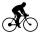Cyclist goes on a trip. First, drove 4 hours average speed 44 kilometers per hour, then slowed down and another 4 hours driving a speed 36 kilometers per hour. How many total kilometers cycling?
3. Winnie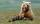Winnie Hugo had the right paw 10 punches and on 15 left more. How many punches has on left paw?
4. Cow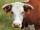104 cow consumes 6968 kg hay per day. How many kg of hay consume one cow in one day?
5. Vinegar 2How many percentage of getvinegar solution, if we mix to 3.5 liters of 5.8% and 5 liters of 7.6% vinegar?
6. Derivation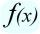Exists a function whose derivation is the same function?
7. SequenceWrite the first 7 members of an arithmetic sequence: a1=-3, d=6.
8. Painter Pavel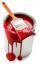Painter Pavel painted the fence for 16 hours and painter Petr the same fence painted for 13 h. How long should take painted the fence together?
9. MilimetersHow many millimeters is 1/4 meters?
10. DigitsWrite the smallest and largest 1-digit number.
11. Cakes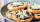On the table was 56 cakes. When Peter went home, leaving them eight times less. How many cakes left?
12. Trapezoid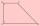Are diagonals in a rectangular trapezoid perpendicular and bisect the angles?
13. DiscountLadies sweater was twice discounted. First by 11%, then by 11% of the new price. Its final price was 100 €. Determine the original price of sweater.Between cities A and B is route 13 km long of stúpanie average 7‰. Calculate the height difference of cities A and B.
15. Computer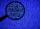A line of print on a computer contains 64 characters (letters, spacers or other chars). Find how many characters there are in 7 lines.
16. Toy Cars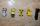Peter has 29 toy cars, Zdenko has 8 toy cars less. How many toy cars has Zdenko?
17. WordWhat is the probability that a random word composed of chars E, Y, G, E, R, O, M, T will be the GEOMETRY?
18. Percent calculationCalculate 61% if 85% is 38.
19. Hours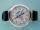How many hours is 9 days?
20. Pizza master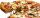Master says that he can splits pizza to 16 parts by five equals straight cuts. Is it possible?

Do you have an interesting mathematical word problem that you can't solve it? Submit math problem, and we can try to solve it.

We will send a solution to your e-mail address. Solved examples are also published here. Please enter the e-mail correctly and check whether you don't have a full mailbox.

Please do not submit problems from current active competitions such as Mathematical Olympiad, correspondence seminars etc...